# Example Code

## Code and Documents

### Attachment

Overview

This VI allows you to convert a single precision number to binary IEEE 754 representation and vice versa.

Description

LabVIEW uses the IEEE 754 standard when rounding a floating point number.  The value of a IEEE-754 number is computed as: sign * 2exponent * mantissa

The sign is stored in bit 32. The exponent can be computed from bits 24-31 by subtracting 127. The mantissa (also known as fraction) is stored in bits 1-23.

This VI provides insight on how the IEEE 754 binary value is calculated.

Requirements

LabVIEW 2012 (or compatible)

Steps to Implement or Execute Code

2. Choose a tab to convert from binary to SGL or vice versa

3. Set your numberor the binary value

4. Run the VI

Block Diagram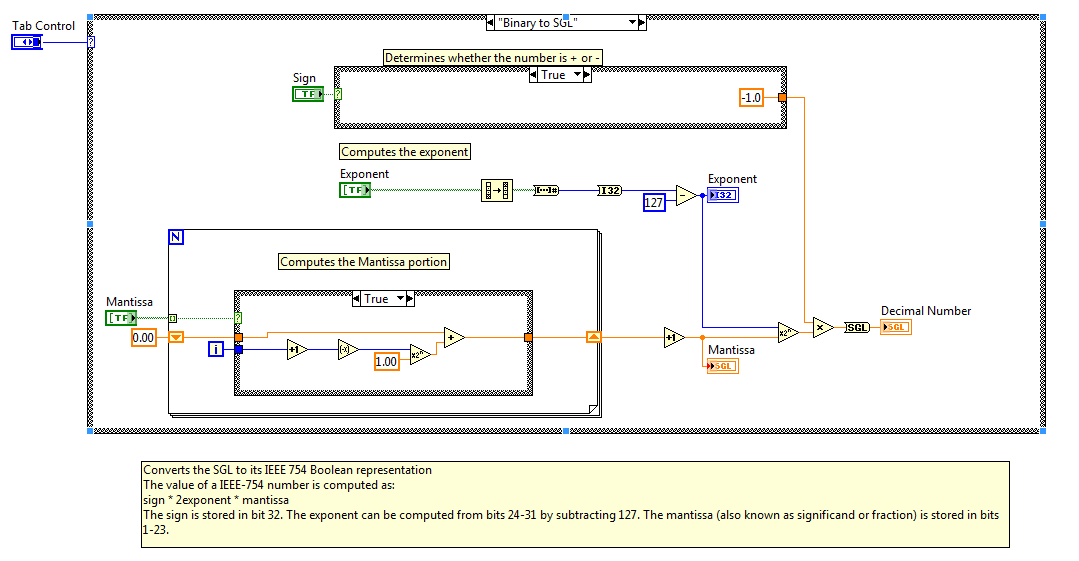Front Panel*This document has been updated to meet the current required format for the NI Code Exchange**

John Passiak

Example code from the Example Code Exchange in the NI Community is licensed with the MIT license.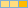Active Participant
on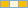Member
on

Simple and useful. Thank you.

Erwin Franz R.

Certified LabVIEW Architect, Certified TestStand Developer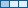Member
on

Very useful code. Thanks.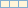Member
on

Hi

The code was really helpful to me, but i could you please tell me how to incorporate storage format into this.

(

For example, I have a floating point number -177.609375 and storage format is s8.7 then i suppose to get HEX  A732 .

I have done code like

EX:1

i took the HEX A732 and storage format s8.7( in the main VI Enter 7 in the f control and in the signed unsigned control write s, in HEX write A732 ) i got the Value as -177.609375 this is valid.

Ex:2

i took the HEX A732 and storage format s15.0 i got the Value as -22734 this is valid as per our requirement.

(I have attached the Code for above examples)

Now by taking DBL value -177.609375 and storage format s8.7 as inputs i the output should be HEX A732

you can find the attached code in the below link

Regards,

NafeesMember
on

This code is really useful but I believe there is a problem in representing a value of zero.

Converting zero to a binary value gives a mantissa of zero and an exponent of 127 which you may expect.

If this is then converted back you get a value of 1 in the mantissa and zero in the exponent which corresponds to 2^0 * 1 = 1.

I can fudge my way around this but would be interested in anyone else's comments.

The instrument I am interfacing with represents zero with a mantissa and exponent of zero which is actually =

0.00000000000000000000000000000000000000587747175411143751 (close enough for me).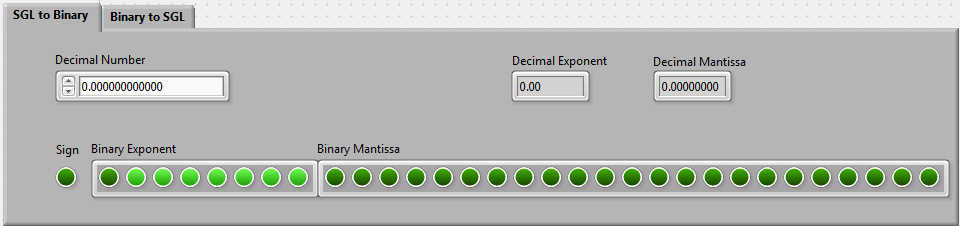Member
on

i know this is an old post but you made a mess of a simple convert that labview do for you  !!!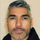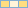Member
on

The conversion is simply a typecast.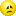Member
on
• Why is the conversion accuracy not very high?Member
on

@Popobbb and rest of thread. I am trying to represent a number that is smaller than the LSB of the exponent bit being set. The number I am feeding into the calculator is 9.18368974565e-41. H-Schmidt IEEE floating point online calculator reports it as denormalized. Can this LV converter handle denormalized values and if it can't can the converter you provided, Popobbb, handle these values.

Thanks.